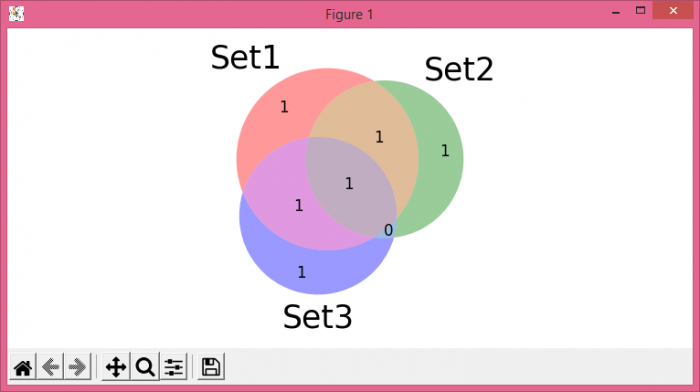# How to modify the font size in Matplotlib-venn?

To modify the font size in Matplotlib-venn, we can use set_fontsize() method.

## Steps

• Set the figure size and adjust the padding between and around the subplots.
• Create three sets for Venn diagram.
• Plot a 3-set area-weighted Venn diagram.
• To set the set_labels and subset_labels fontsize, we can use set_fontsize() method.
• To display the figure, use show() method.

## Example

from matplotlib import pyplot as plt
from matplotlib_venn import venn3

plt.rcParams["figure.figsize"] = [7.50, 3.50]
plt.rcParams["figure.autolayout"] = True

set1 = {'a', 'b', 'c', 'd'}
set2 = {'a', 'b', 'e'}
set3 = {'a', 'd', 'f'}

out = venn3([set1, set2, set3], ('Set1', 'Set2', 'Set3'))

for text in out.set_labels:
text.set_fontsize(25)

for text in out.subset_labels:
text.set_fontsize(12)

plt.show()

## Output